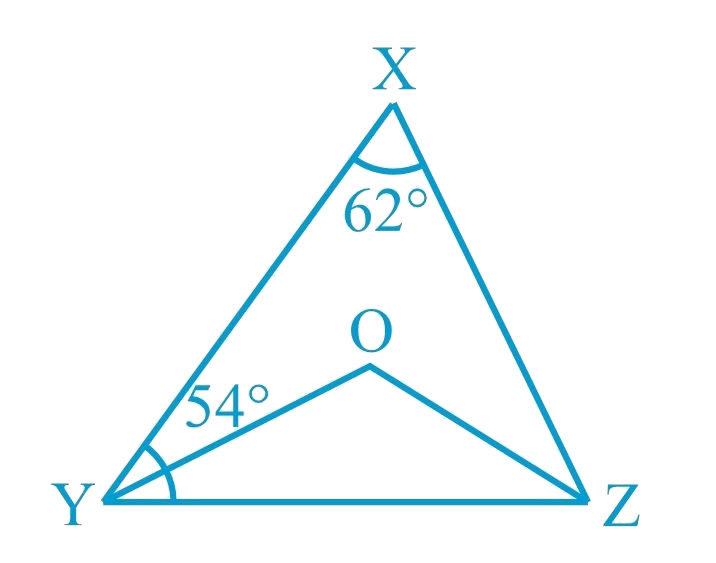# Ex.6.3 Q2 Lines and Angles Solution - NCERT Maths Class 9

## Question

In Fig. below, $$\angle X = 62^\circ ,{\rm{ }}\angle XYZ = 54^\circ$$. If $$YO$$ and $$ZO$$ are the bisectors of $$\angle XYZ$$ and $$\angle XZY$$ respectively of $$\Delta XYZ,$$ find $$\angle OZY$$ and $$\angle YOZ$$ .## Text Solution

What is known?

$$\angle X = 62^\circ ,{\rm{ }}\angle XYZ = 54^\circ$$ and $$YO$$ and $$ZO$$ are the bisectors of $$\angle XYZ$$ and $$\angle XZY$$ respectively.

What is unknown?

$$\angle OZY$$ and $$\angle YOZ$$

Reasoning:

As we know the angle sum property of a triangle:

Sum of the interior angles of a triangle is $$360^\circ$$.

Steps:

Given, in $$\Delta XYZ$$,

\begin{align}\angle X &= 62^\circ \\\angle XYZ &= 54^\circ \end{align}

\begin{align}\angle X + \angle XYZ + \angle Z &= 180^\circ\\ ( \text{Angle sum property of }&\text{a triangle}\rm{.} )\\\\62^\circ + {\rm{ 5}}4^\circ + \angle Z &= 180^\circ \\\angle Z &=180^\circ-116^\circ \\\angle Z &= 64^\circ \end{align}

Now, $$OZ$$ is angle bisector of $$\angle XZY$$

\begin{align} \angle OZY = \frac{1}{2}\angle XZY &= \frac{1}{2} \times 64^\circ \\&= 32^\circ {\rm{ }}\left( {\rm{i}} \right)\end{align}

Similarly, $$OY$$ is angle bisector of $$\angle XYZ$$

\begin{align} \angle OYZ = \frac{1}{2}\angle XYZ &= \frac{1}{2} \times 54^\circ \\ &= 27^\circ \left( {{\rm{ii}}} \right)\end{align}

Now, in $$\Delta OYZ$$

\begin{align}\angle OYZ \!+\! \angle OZY \!+\! \angle YOZ &= 180^\circ \\( \text{Angle sum property of a }&\text{triangle}\rm{.} )\\\\27^\circ +32^\circ + \angle YOZ & = 180^\circ \\\left[ {{\textrm{from }}\left( {\rm{i}} \right){\rm{ and }}\left( {{\rm{ii}}} \right)} \right]\\\\\angle YOZ &\!= 180^\circ \!- 59^\circ \\\angle YOZ &= 121^\circ \end{align}

Hence, $$\angle OZY = 32^\circ$$ and $$\angle YOZ = 121^\circ$$

Learn from the best math teachers and top your exams

• Live one on one classroom and doubt clearing
• Practice worksheets in and after class for conceptual clarity
• Personalized curriculum to keep up with school